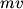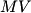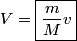## Solution to 1986 Problem 44

 The initial momentum is$mv$ to the right. The final momentum is$MV$ to right. Therefore,\begin{align*}V = \boxed{\frac{m}{M}v}\end{align*}So, answer (A) is correct.